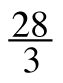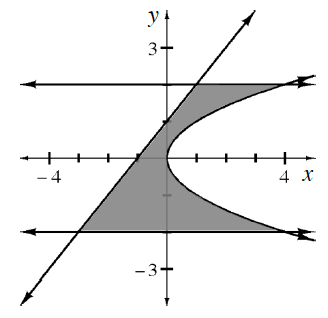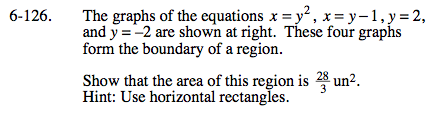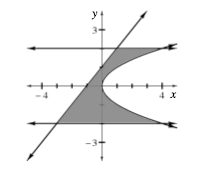### Home > CALC > Chapter 6 > Lesson 6.4.1 > Problem6-126

6-126.
1. The graphs of the equations x = y 2, x = y −1, y = 2, and y = −2 are shown below. These four graphs form the boundary of a region. Homework Help ✎

2. Show that the area of this region isun2. Hint: Use horizontal rectangles.

3.dy:
Since we are using horizontal rectangles, the infinitely small widths of each rectangle are measured on the y-axis...
hence we us dy instead of dx.

The bounds:
Notice that BOTH equations are written with y as the input and x as the output.
Therefore, find the bounds of integration will be on the y-axis.
A = lower y value
B = upper y value

The integrand:
The 'top function' and 'bottom function' will also be determined with respect to the y-axis.
Let the 'top function' be the one with the right-most position, while the 'bottom function' will be the one with the 'left-most position.'
Another way to look at this is the 'top function' has the highest x-values, while the 'bottom function' has the lower x-values.